Updating search results...

# 38 Results

View
Selected filters:
• Look At Physics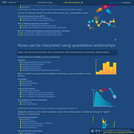Conditional Remix & Share Permitted
CC BY-SA
Rating
0.0 stars

In the first part of this video, we derive the law of mass action from one example of a picture of molecular collisions. For this course, we use the "law of mass action" to refer to an idea that chemical reaction kinetic rates can be expressed using products of the abundances of reactants raised to exponents. Studying cooperativity and Hill functions in the second part of the video allows us to investigate a simple example of bistability in the third video segment.

Subject:
Chemistry
Life Science
Mathematics
Physical Science
Physics
Material Type:
Lecture Notes
Provider:
Look At Physics
Provider Set:
A Mathematical Way to Think About Biology
Author:
David Liao
10/08/2012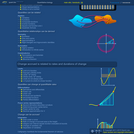Conditional Remix & Share Permitted
CC BY-SA
Rating
0.0 stars

The purpose of this video tutorial is to review a couple ways in which we think about numbers. Thinking in terms of street numbers, money in bank accounts, and quantum particles (e.g. Bose-Einstein condensate) is contrasted with focusing on associating numbers with distinguishable manipulatives, as is more familiar in K-8 courses. This video concludes with a reminder that the symbol "infinity" is not, itself, a number.

Subject:
Mathematics
Physical Science
Physics
Material Type:
Lecture Notes
Provider:
Look At Physics
Provider Set:
A Mathematical Way to Think About Biology
Author:
David Liao
10/08/2011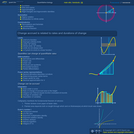Conditional Remix & Share Permitted
CC BY-SA
Rating
0.0 stars

When a function depends on multiple independent variables, the curly-d symbol denotes slopes calculated by jiggling only one independent variable at a time. This is a multivariable cousin to the derivative. We use this notation in future sections to keep track of how molecules are generated or degraded by different reactions.

Subject:
Mathematics
Material Type:
Lecture Notes
Provider:
Look At Physics
Provider Set:
A Mathematical Way to Think About Biology
Author:
David Liao
10/08/2012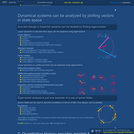Conditional Remix & Share Permitted
CC BY-SA
Rating
0.0 stars

Why do quantitative biologists sometimes claim that mRNA copy numbers are Poisson distributed in simple models of gene transcription? The first video segment addresses this question under the simplifying assumption that mRNA degradation occurs after a well-defined, deterministic lifetime, and the second segment illustrates the same basic concept for the more realistic situation in which degradation is stochastic.

Subject:
Life Science
Mathematics
Material Type:
Lecture Notes
Provider:
Look At Physics
Provider Set:
A Mathematical Way to Think About Biology
Author:
David Liao
10/08/2013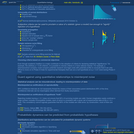Conditional Remix & Share Permitted
CC BY-SA
Rating
0.0 stars

After heuristically deriving Stirling's approximation in the first video segment, we outline a simple example of the central limit theorem for the case of the binomial distribution. In the final segment, we explain how the central limit theorem is used to suggest that physical experiments are characterized by normally-distributed (Gaussian) fluctuations while fluctuations in biological experiments are said to fill out log-normal distributions.

Subject:
Life Science
Mathematics
Physical Science
Physics
Material Type:
Lecture Notes
Provider:
Look At Physics
Provider Set:
A Mathematical Way to Think About Biology
Author:
David Liao
10/08/2012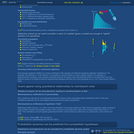Conditional Remix & Share Permitted
CC BY-SA
Rating
0.0 stars

In the first video segment, we study the distribution, average, and variance for the Bernoulli coin-toss process. The binomial distribution results from stringing together a series of coin tosses. In the second segment, we study the limit of "rare" events, which is described by the Poisson distribution.

Subject:
Mathematics
Material Type:
Lecture Notes
Provider:
Look At Physics
Provider Set:
A Mathematical Way to Think About Biology
Author:
David Liao
10/08/2012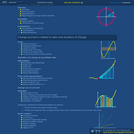Conditional Remix & Share Permitted
CC BY-SA
Rating
0.0 stars

The quadratic formula is easy to solve, yet sufficiently sophisticated that it provides insight into oscillations of masses connected by springs, as well as insight into chemical bonds between atoms. The purpose of this video is to illustrate what it means to find the "zeros" or "roots" of the quadratic equation, both using a graphical description, as well as by analytically completing the square to obtain the famous quadratic formula.

Subject:
Mathematics
Material Type:
Lecture Notes
Provider:
Look At Physics
Provider Set:
A Mathematical Way to Think About Biology
Author:
David Liao
10/08/2011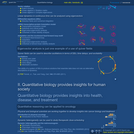Conditional Remix & Share Permitted
CC BY-SA
Rating
0.0 stars

We use eigenvector-eigenvalue analysis to walk through a simple quasispecies model described in Bull, Meyers, and Lachmann, "Quasispecies made simple," PLoS Comp Biol, 1(6):e61 (2005). The dominance of a genotype depends, not merely on its ability to breed quickly (i.e. the rudimentary concept of survival of the fittest), but also on its ability to breed "true."

Subject:
Ecology
Forestry and Agriculture
Geoscience
Life Science
Mathematics
Physical Science
Material Type:
Lecture Notes
Provider:
Look At Physics
Provider Set:
A Mathematical Way to Think About Biology
Author:
David Liao
10/08/2012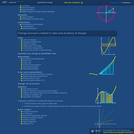Conditional Remix & Share Permitted
CC BY-SA
Rating
0.0 stars

When a derivative of a function appears on one side of an equation and the function appears somewhere on the other side, it is common to employ a series of manipulations of notation that are called separation of variables. This strategy is often presented in a way that makes it seem as though differentials were quantities that could be independently moved around an equation. The purpose of this video is to show that the same end result can be obtained more rigorously using u-substitution, in other words, using a "change of variables."

Subject:
Mathematics
Material Type:
Lecture Notes
Provider:
Look At Physics
Provider Set:
A Mathematical Way to Think About Biology
Author:
David Liao
10/08/2011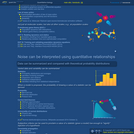Conditional Remix & Share Permitted
CC BY-SA
Rating
0.0 stars

In a toy model of a cell, protein X is produced according to a translation rate coefficient and eliminated according to a degradation rate coefficient. The protein copy number at which the rates for these processes balance is called the steady-state level, and the time it takes for a cell initially containing zero copies of protein X to accumulate half the steady-state level is called the _ŃŇrise time._Ń Surprisingly, the "rise time" depends on the degradation rate coefficient only. The classic textbook presentation of this topic is found in Alon, An Introduction to Systems Biology: Design Principles of Biological Circuits, Boca Raton: Chapman & Hall/CRC, 2007 (p. 18-22).

Subject:
Life Science
Mathematics
Material Type:
Lecture Notes
Provider:
Look At Physics
Provider Set:
A Mathematical Way to Think About Biology
Author:
David Liao
10/08/2012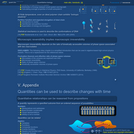Conditional Remix & Share Permitted
CC BY-SA
Rating
0.0 stars

In the first video segment, we describe the fundamental postulate of statistical mechanics. The direct product notation we introduce in the second segment helps us to discuss the states available to a collection of many parts, which helps us, in turn, to derive the Boltzmann factor in the third segment. The fourth video segment explains how the Boltzmann factor helps us to calculate average properties for systems in thermal contact with large baths and introduces entropy (Greek letter sigma), free energy (F), and the partition function (Z).

Subject:
Chemistry
Mathematics
Physical Science
Physics
Material Type:
Lecture Notes
Provider:
Look At Physics
Provider Set:
A Mathematical Way to Think About Biology
Author:
David Liao
10/08/2012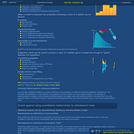Conditional Remix & Share Permitted
CC BY-SA
Rating
0.0 stars

Students will encounter the concept of a distribution, along with parameters that describe a distribution's "typical" values (average) and a distribution's spread (variance). To understand simple distributions and uncertainty propagation in the coming sections, it is necessary to be familiar with the concept of statistical independence. When two variables fluctuate independently, their covariance vanishes, and the variance of their sum is the sum of their variances.

Subject:
Mathematics
Material Type:
Lecture Notes
Provider:
Look At Physics
Provider Set:
A Mathematical Way to Think About Biology
Author:
David Liao
10/08/2012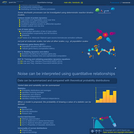Conditional Remix & Share Permitted
CC BY-SA
Rating
0.0 stars

Even when we model the dynamics of the abundances of molecules inside biological systems using calculus, it is important to remember that underlying behavior can be apparently random ("stochastic"). Even a deterministic system containing components moving in periodic ways can, at early times, support dynamics that appear disordered. The behavior of systems containing complicated collections of interacting parts can be difficult to predict with accuracy (chaos). Finally, systems can display stochasticity because the outcomes of measurements on quantum systems are indeterminate in a fundamental way. Random processes are modeled using Markov models.

Subject:
Life Science
Mathematics
Physical Science
Physics
Material Type:
Lecture Notes
Provider:
Look At Physics
Provider Set:
A Mathematical Way to Think About Biology
Author:
David Liao
10/08/2012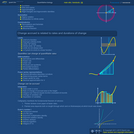Conditional Remix & Share Permitted
CC BY-SA
Rating
0.0 stars

The purpose of the first part of this video is to introduce the idea of summation and its notation using the Greek-letter Sigma. We practice working with sums by using Gauss's summation trick. In the second part of the video, we study examples of infinite series, one which converges to a finite number (geometric series), and one which diverges (harmonic series).

Subject:
Mathematics
Material Type:
Lecture Notes
Provider:
Look At Physics
Provider Set:
A Mathematical Way to Think About Biology
Author:
David Liao
10/08/2012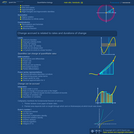Conditional Remix & Share Permitted
CC BY-SA
Rating
0.0 stars

To continue our discussion of derivatives from preceding videos, we explain that the second derivative represents curvature. By combining knowledge of multiple derivatives, we can sometimes create Taylor series, which are local approximations of functions. As an example, we Taylor-expand sinusoidal functions and then use the results to iteratively approximate pi.

Subject:
Mathematics
Material Type:
Lecture Notes
Provider:
Look At Physics
Provider Set:
A Mathematical Way to Think About Biology
Author:
David Liao
10/08/2011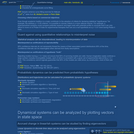Conditional Remix & Share Permitted
CC BY-SA
Rating
0.0 stars

Students will learn a sample-variance curve fitting method that can be used to determine whether a set of experimental data appears to have been generated by a model. This method is based on minimizing the reduced chi-squared value. This video includes a reminder to inspect normalized residuals before reporting fitted parameters.

Subject:
Mathematics
Physical Science
Physics
Material Type:
Lecture Notes
Provider:
Look At Physics
Provider Set:
A Mathematical Way to Think About Biology
Author:
David Liao
10/08/2012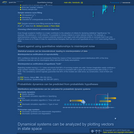Conditional Remix & Share Permitted
CC BY-SA
Rating
0.0 stars

The quadrature formula relates the fluctuations of a function to fluctuations in the variables on which the function depends. In this derivation, we approximate a multivariable function using a Taylor expansion, and we assume that fluctuations in the underlying variables are statistically independent, which allows us to apply an identity previously derived in the unit on statistics. Namely, "variances of sums are sums of variances" for variables that fluctuate independently.

Subject:
Mathematics
Physical Science
Physics
Material Type:
Lecture Notes
Provider:
Look At Physics
Provider Set:
A Mathematical Way to Think About Biology
Author:
David Liao
10/08/2012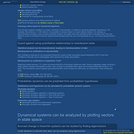Conditional Remix & Share Permitted
CC BY-SA
Rating
0.0 stars

In the first video segment, we estimate properties of a parent distribution (i.e. mean and standard deviation) from a sample of a finite collection of data measurements, and we describe the standard error (SE). In the second segment, we derive the famous square-root of n factor that appears in the SE formula. The third video segment describes the visual comparison of error bars, and the fourth segment warns against a mistake that can generate inappropriate claims of statistical significance during this kind of analysis.

Subject:
Life Science
Mathematics
Physical Science
Physics
Material Type:
Lecture Notes
Provider:
Look At Physics
Provider Set:
A Mathematical Way to Think About Biology
Author:
David Liao